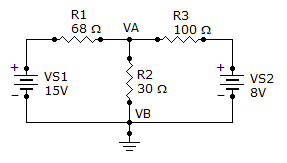# Electrical Engineering - Branch, Loop and Node Analyses - Discussion

Discussion Forum : Branch, Loop and Node Analyses - General Questions (Q.No. 2)
2.
What is the current through R2?3.19 A
319 mA
1.73 A
173 mA
Explanation:
No answer description is available. Let's discuss.
Discussion:
16 comments Page 1 of 2.

Jaywonder said:   6 years ago
Can anyone find the answer using Thevenin's theorem?

Umair said:   7 years ago
@Somashekhar.

How you got that 30096? Explain.

Krishna said:   8 years ago
The answer must me same irrespective of method we acquire but I did't get answer using mesh analysis.

Akinremi Akinola Musbau said:   8 years ago
@Shinghal.

Your working is right but take note that the current through 30 ohms is the sum of the current that emanate from the two cell and not the difference.

Kavan Rathod said:   8 years ago
Simple apply node analysis and solve it.

Somashekhar y said:   8 years ago
((va-12)/49)+(va/24)+((va-6)/80) = 0.
((va-12)(1920)+(va)(3920)+(va-6)(1176))/94080 = 0.

va(7016)-30096 = 0.
va = 30096/7016.
va = 4.28.

Please review previous question. Why nodal equation differs? Can any one explain?

Pente vinodkumar said:   8 years ago
By applying loop analysis at first loop.

15 = 68i'+ 30(i'+i").
15 = 98i'+ 30i".

Now at second loop:

8 = 100i"+30*(i'+i").
8 = 30i'+130i".

After calculation:

i' = 0.145.
i" = 0.028.

So total current in 30 ohm resistance.

i' + i".

0.145 + 0.028.

= 173 mA.
(2)

Julius said:   9 years ago
Can you please elaborate the solution so that I can understand. Thanks.

Sunilkumar v said:   1 decade ago
Apply nodal analysis for the circuit. After getting Va value, we can find current at 30 ohm.res

(15-VA)/68 - (VA-0)/30 - (VA-8)/100 = 0.

VA = 5.169.

R2 = VA/30.

R2 = 5.169/30.

R2 = 173 mA.
(1)

Dinesh kumar t said:   1 decade ago
Applying kcl at node A and taking node B as refrence:

(15-va)/68-va/30-(va-8)/100=0

va=5.1689v;

Ir2=va/30=5.1689/30=0.172A=172mA.